### A more probabilistic view on multiple comparisons problem

Even though this blog is not going to be only about multiple comparisons (I could not think of another name), I decided to write about an old problem in slightly new way.

# Multiple Comparisons

Whenever we are testing many hypotheses and are trying to figure out which of them are true we stumble upon so called Multiple Comparisons problem. This is especially evident in fields where we do tens of thousands tests (such as neuroimaging or genetics). So what is the big deal? Imagine that you divide the brain into a buch of regions (voxels) and for each of them you will perform some statistical test (checking for example if this part of the brain is involved in perception of kittens). Some of the regions will yield high statistical values (suggesting relation to kittens) and some will not. Lets try to show this with a simple simullation.

Let's assume for now that we will test 100 voxels and only 10 of them will be related to kittens. We will model both populations of voxels using Gaussian distributions. Noise distribution will be centred on zero opposed to signal centred on three.

In :
```import numpy as np
noise_voxels = np.random.normal(size=90, loc=0.0, scale=1.0)
signal_voxels = np.random.normal(size=10, loc=3.0, scale=1.0)
```

Lets plot this

In :
```import pylab as plt
figsize(10,6)
plt.hist([noise_voxels, signal_voxels], bins=20, label=['noise', 'kittens'], histtype='step', fill=True, stacked=True)
plt.legend()
```
Out:
`<matplotlib.legend.Legend at 0x105eb9790>`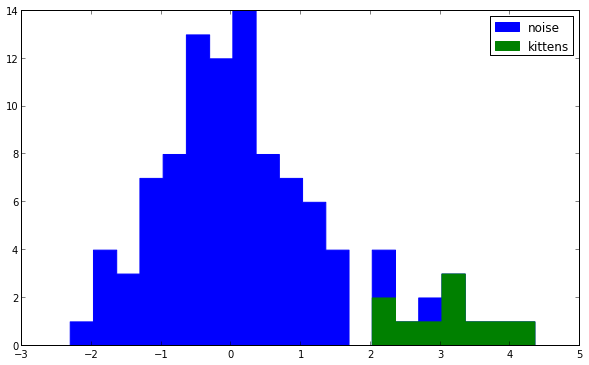Even though noise is dominating in this example it would be very easy to draw a line distinguishing non-kitten related voxels from those that really do say "meow". What does it has to do with multiple comparisons will be clear in a moment.

Firstly let's show that this is just a simulation and depending on what mood my computer is in the results will be different. Here are four instances.

In :
```for i in range(4):
plt.subplot(2,2,i)
noise_voxels = np.random.normal(size=90, loc=0.0, scale=1.0)
signal_voxels = np.random.normal(size=10, loc=3.0, scale=1.0)
plt.hist([noise_voxels, signal_voxels], bins=20, label=['noise', 'kittens'], histtype='step', fill=True, stacked=True)
plt.legend()
```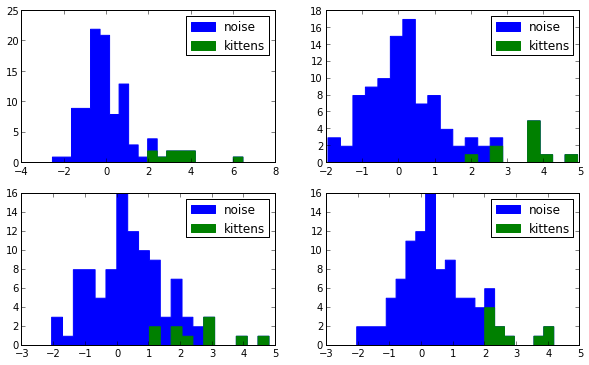We can operate on the theoretical distributions instead of just the simulations. Since we are dealing with two Gaussians let's plot two Gaussians.

In :
```x_range = np.linspace(-3,6,100)
noise_samples = 90.0
signal_samples = 10.0
snr = signal_samples/noise_samples
from scipy.stats import norm
plt.plot(x_range, norm.pdf(x_range)*(1-snr), 'b', label="noise")
plt.plot(x_range, norm.pdf(x_range,loc=3)*(snr), 'g', label="kittens")
plt.legend()
```
Out:
`<matplotlib.legend.Legend at 0x105b7ac50>`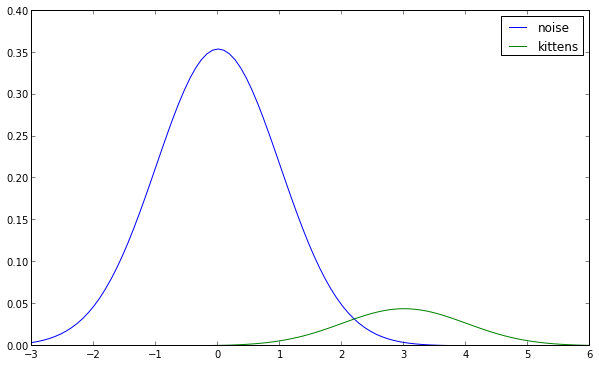Now we can clearly see that the overlap between the two distributions is fairly small. Notice that there are two important parameters that can influence this: Signal to Noise Ration (SNR) and location of the signal distribution (also known as the effect size).

The multiple comparisons problem is all about... well multiple comparisons so in other words the number of tests we make. In our example this is equivalent to how many voxels we have). So let's show this by upsampling our data! Let's say we will be able to divide each old (big) voxel into eight small voxels.

In :
```noise_samples = 90.0*8
signal_samples = 10.0*8
snr = signal_samples/noise_samples
plt.plot(x_range, norm.pdf(x_range)*(1-snr), 'b', label="noise")
plt.plot(x_range, norm.pdf(x_range,loc=3)*(snr), 'g', label="kittens")
plt.legend()
```
Out:
`<matplotlib.legend.Legend at 0x10756a150>`Surprisingly nothing has changed... But we have more voxels and did more comparisons (tested more hypotheses)! True, but becaue we only upsampled the data we just created identical copies of old values. SNR thus stayed the same. However, things change when we consider a more realistic situation than "10% of the brain selectively responds to young cats". Out of 60000 voxels (average head size, 4x4x4mm resolution, skull stripped) 100 will respond to kittens.

In :
```noise_samples = 60000.
signal_samples = 100.
snr = signal_samples/noise_samples
plt.plot(x_range, norm.pdf(x_range)*(1-snr), 'b', label="noise")
plt.plot(x_range, norm.pdf(x_range,loc=3)*(snr), 'g', label="kittens")
plt.legend()
```
Out:
`<matplotlib.legend.Legend at 0x1093126d0>`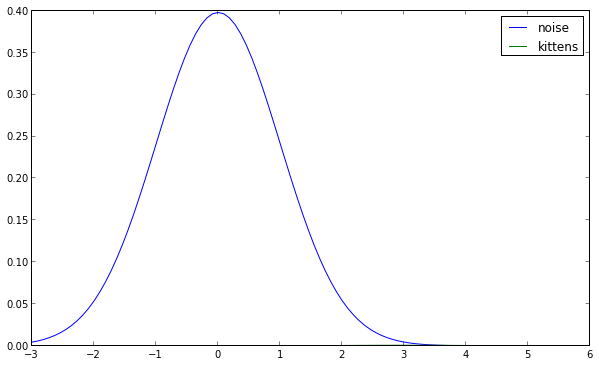Where are the cats gone?!? Let's have a closer look.

In :
```plt.plot(x_range, norm.pdf(x_range)*(1-snr), 'b', label="noise")
plt.plot(x_range, norm.pdf(x_range,loc=3)*(snr), 'g', label="kittens")
plt.legend()
plt.xlim([0,6])
plt.ylim([0.00,0.01])
```
Out:
`(0.0, 0.01)`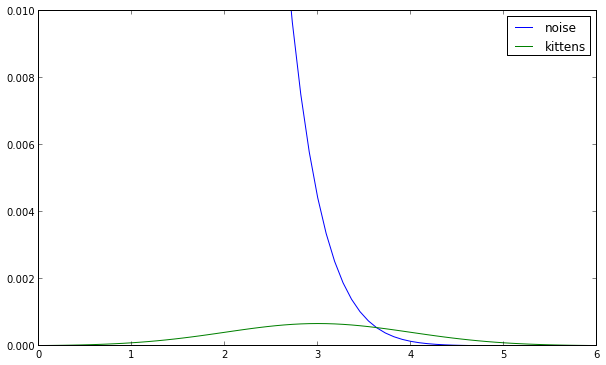Haha! If we zoom in we will be able to find the signal distribution dwarfed by the noise. The problem is not the number of comparison we do but the fraction of those comparison that will be yield no signal. If you look carefully you will notice that the crossing point between the distributions increased with decreased SNR. This crossing is a potential candidate for a threshold. Let's try to find this point.

In :
```from scipy.optimize import fsolve
fsolve(lambda x : norm.pdf(x)*(1-snr) - norm.pdf(x, loc=3)*(snr),2.0)
```
Out:
`array([ 3.26443494])`

The interesting aspect is the relation between this crossing point and SNR.

In :
```snrs = np.linspace (0.3,0.005, 1000)
crossing_points = []
for snr in snrs:
crossing_point = fsolve(lambda x : norm.pdf(x)*(1-snr) - norm.pdf(x, loc=3)*(snr),2.0)
crossing_points.append(crossing_point)

plt.plot(snrs, crossing_points)
plt.xlabel("SNR")
plt.ylabel("crossing point")
```
Out:
`<matplotlib.text.Text at 0x109629110>`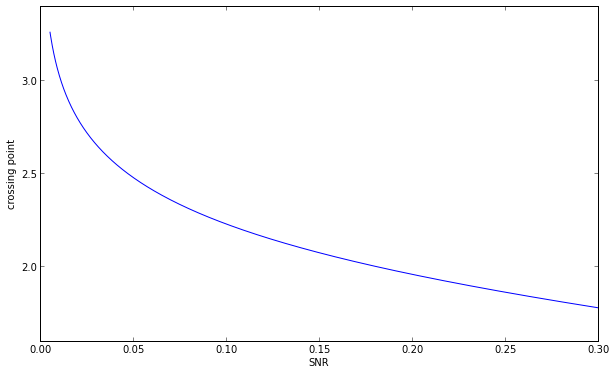As we can see it reises sharply for very small SNR values. Another popular option for picking a threshold is controling for False Discovery Rate. The fraction of false discoveries among all voxels labeled as significant. This is equivalent to the ratio of area under the blue curve right of the threshold to the sum of the areas under the blue and green curves right of the threshold. This areas are summarized by the Cumulative Distribution Functions (CDFs).

In :
```thr = 3.26
(1-norm.cdf(thr))*(1-snr)/((1-norm.cdf(thr))*(1-snr) + (1-norm.cdf(thr, loc=3))*snr)
```
Out:
`0.45640041955468291`

Another important value is the percentage of missed voxels.

In :
```norm.cdf(thr, loc=3)
```
Out:
`0.6025681132017604`

As mentioned before a popular option in dealing with Multiple Comparisons is to keep the FDR at a certain level (usually 0.05). Let's see what happens to percentage of missed voxels if we do this at different SNRs.

In :
```missed_voxels = []
fdr_thresholds = []
for snr in snrs:
fdr_thr = fsolve(lambda x : (1-norm.cdf(x))*(1-snr)/((1-norm.cdf(x))*(1-snr) + (1-norm.cdf(x, loc=3))*snr)-0.05,2.0)
missed_voxels.append(norm.cdf(fdr_thr, loc=3))
fdr_thresholds.append(fdr_thr)

plt.plot(snrs, missed_voxels)
plt.xlabel("SNR")
plt.ylabel("percentage of missed voxels")
plt.figure()
plt.plot(snrs, fdr_thresholds)
plt.xlabel("SNR")
plt.ylabel("FDR corrected threshold")
```
Out:
`<matplotlib.text.Text at 0x10966df90>`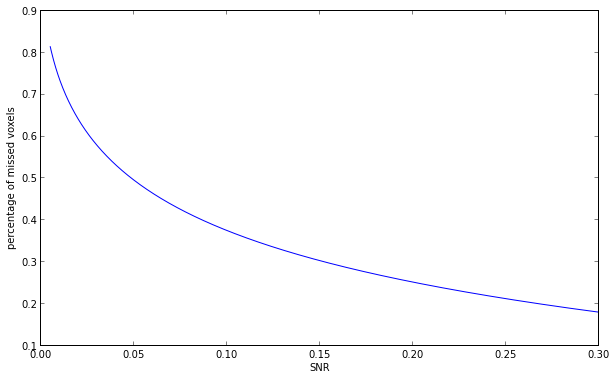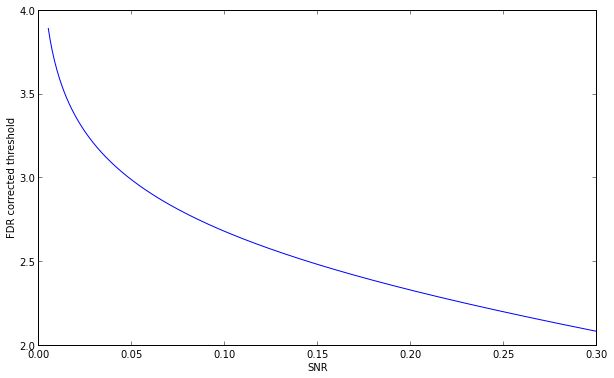From this plot we can see that when we decrease SNR, even though we control for FDR we are missing a lot of voxels. For extremely low SNR and small absolute number of signal voxels chances of finding anything are very slim.

# Take home message

In this inaugral post I was trying to show multiple comparison problem in a slightly different view. I hope that from those simple simulations it will be clear that the problem is not really about the number of tested hypotheses, but the ration between noise and signal. Next week I'll try to write about something more light hearted :)

1.